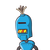# What is the sum of the successor of the smallest 3 -digit number and the predecessor of the largest 4-digit number?2 p

What is the sum of the successor of the smallest 3 -digit number and the predecessor of the largest 4-digit number?

2 points

10,099

10,009

11,009

10,999​

### 1 thought on “What is the sum of the successor of the smallest 3 -digit number and the predecessor of the largest 4-digit number?<br /><br />2 p”

1.10,099

Step by Step Solution

hey friend here is your solution

The smallest 3-digit number is 100

then, successor of 100 = 100+1= 101

The largest 4-digit is 9999

then, predecessor of 9999= 9999-1= 9998

There sum is

101+9998 = 10,099

I hope it’s helpful for you